AP State Syllabus AP Board 7th Class Maths Solutions Chapter 8 Congruency of Triangles Ex 4 Textbook Questions and Answers.

## AP State Syllabus 7th Class Maths Solutions 8th Lesson Congruency of Triangles Exercise 4Question 1.
Which congruence criterion do you use in the following?
(i) Given :AC = DF
AB = DE
BC = EF
So, ΔABC ≅ ΔDEF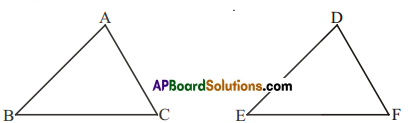(ii) Given: ZX = RP
RQ = ZY
∠PRQ ≅ ∠XZY
So, ΔPQR ≅ ΔXYZ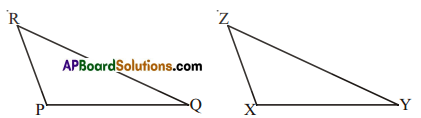(iii) Given: ∠MLN ≅∠FGH
∠NML ≅ ∠GFH
ML = FG
So, ΔLMN ≅ ΔGFH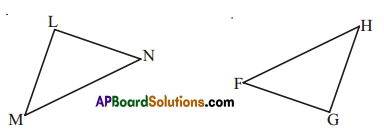(iv) Given: EB = DB . D
AE = BC
∠A = ∠C = 90°
So, ΔABE ≅ ΔCDB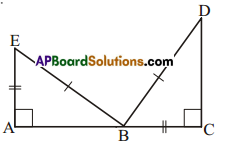Solution:
(i) S.S.S congruence
(ii) S.A.S congruence
(iii) A.S.A congruence
(iv) By R.H.S congruenceQuestion 2.
You want to show that ΔART ≅ ΔPEN,
(i) If you have to use SSS criterion, then you need to show
(a) AR= (b) RT = (c) AT=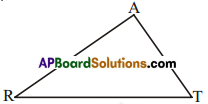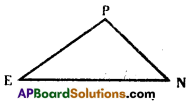Solution:
(i) (a) AR = PE
(b) RT = EN
(c) AT = PN

(ii) If it is given that ∠T = ∠N and you are to use SAS criterion, you need to have
(a) RT = ? and (ii) PN = ?
Solution:
(a) RT = EN and
(ii) PN = AT

(iii) If it is given that AT = PN and you arelo use ASA criterion, you need to have
(a)? (b)?
Solution:
a) ∠A = ∠P
b) ∠T = ∠NQuestion 3.
You have to show that ΔAMP ≅ ΔAMQ. In the following proof. supply the missing reasons.

 Steps Reasons (i) PM = QM (i) ……………. (ii) ∠PMA ≅ ∠QMA (ii) ……………. (iii) AM = AM (iii) ……………. (iv) ∆AMP ≅ ∆AMQ (iv) …………….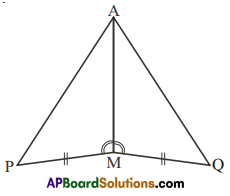Solution:

 Steps Reasons (i) PM=QM (i) Given side (ii) ∠PMA ≅ ∠QMA (ii) Given angle (iii) AM=AM (iii) Common side (iv) ∆AMP ≅ ∆AMQ (iv) A.A.S criterionQuestion 4.
In ΔABC’, ∠A = 30°, ∠B = 40° and ∠C = 110°
In ΔPQR, ∠P = 30°, ∠Q = 40°and ∠R = 110°
A student says that ΔABC ≅ ΔPQR by AAA congruence criterion. Is hejustified? Why or why not?
Solution:
The student Is not correct.
AAA is not a congruency criteria.
Triangles with same corresponding angles can have different sizes.

Question 5.
In the figure, the two triangles are congruent. The corresponding parts are marked. We can write ΔRAT? ≅ ?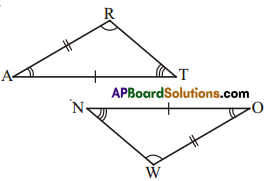Solution:
ΔRAT ≅ ΔWON

Question 6.
Complete the congruence statement.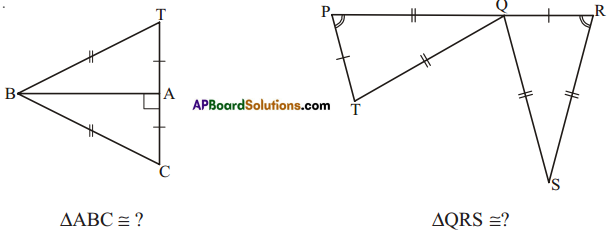Solution:
ΔABC ≅ ?
ΔABC ≅ ΔABT

ΔQRS ≅ ?
ΔQRS ≅ ΔTPQQuestion 7.
In a squared sheet, draw two triangles of equal areas such that
(i) the triangles are congruent.
(ii) the triangles are not congruent.
What can you say about their perimeters?
Solution: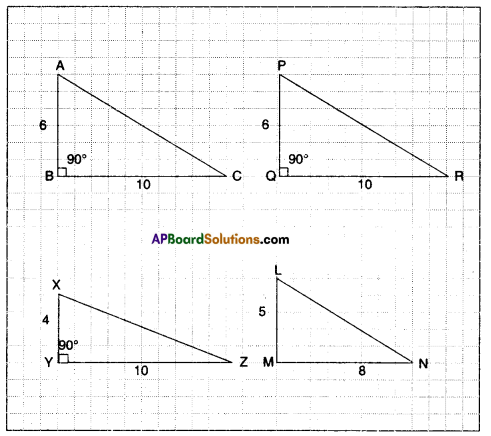i) ΔABC ≅ ΔPQR
Since AB = PQ
∠B = ∠Q
BC = QR (Perimeter is same)

ii) Area of ΔXYZ = $$\frac { 1 }{ 2 }$$ x 10 x 4 : 20 sq.units.
Areas of ΔLMN= $$\frac { 1 }{ 2 }$$ x 8 x 5=2Osq. units
But ΔXYZ and ΔLMN are not congruent.
(Perimeter is different)

Question 8.
If ΔABC and ΔPQR are to be congruent, name one additional pair of corresponding parts. What criterion did you use?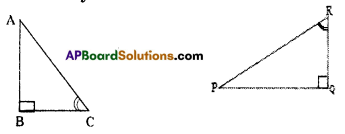Solution:
We need to know that BC = QR. Here we use A.S.A criterion.

Question 9.
Explain, why
ΔABC ≅ ΔFED.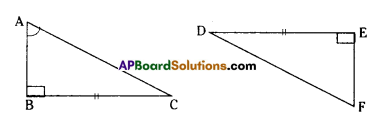Solution:
∠B = ∠E
BC = ED
∠C = ∠D by angle sum property.